# Joins¶

Joins are essential operations in relational databases. They create a link between rows based on common values and allow the meaningful combination of these rows. CrateDB supports joins and due to its distributed nature allows you to work with large amounts of data.

In this document we will present the following topics. First, an overview of the existing types of joins and algorithms provided. Then a description of how CrateDB implements them along with the necessary optimizations, which allows us to work with huge datasets.

## Join types¶

A join is a relational operation that merges two data sets based on certain properties. Join Types (Inspired by this article) shows which elements appear in which join.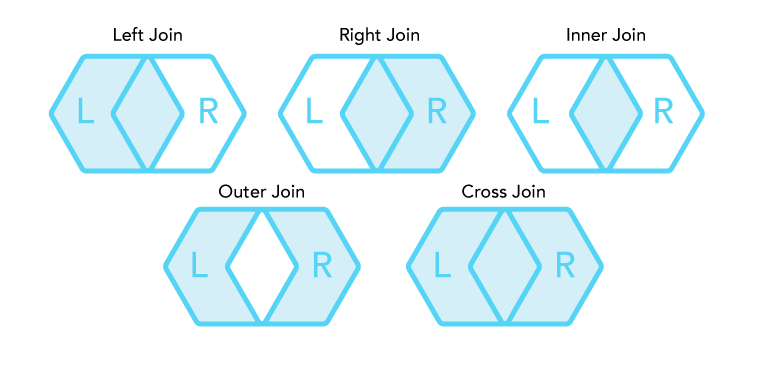Join Types

From left to right, top to bottom: left join, right join, inner join, outer join, and cross join of a set L and R.

### Cross join¶

A cross join returns the Cartesian product of two or more relations. The result of the Cartesian product on the relation L and R consists of all possible permutations of each tuple of the relation L with every tuple of the relation R.

### Inner join¶

An inner join is a join of two or more relations that returns only tuples that satisfy the join condition.

#### Equi Join¶

An equi join is a subset of an inner join and a comparison-based join, that uses equality comparisons in the join condition. The equi join of the relation L and R combines tuple l of relation L with a tuple r of the relation R if the join attributes of both tuples are identical.

### Outer join¶

An outer join returns a relation consisting of tuples that satisfy the join condition and dangling tuples from both or one of the relations, respectively to the outer join type.

An outer join can be one of the following types:

• Left outer join returns tuples of the relation L matching tuples of the relation R and dangling tuples of the relation R padded with null values.

• Right outer join returns tuples of the relation R matching tuples of the relation L and dangling tuples from the relation L padded with null values.

• Full outer join returns matching tuples of both relations and dangling tuples produced by left and right outer joins.

## Join algorithms¶

CrateDB supports (a) CROSS JOIN, (b) INNER JOIN, (c) EQUI JOIN, (d) LEFT JOIN, (e) RIGHT JOIN and (f) FULL JOIN. All of these join types are executed using the nested loop join algorithm except for the Equi Joins which are executed using the hash join algorithm. Special optimizations, according to the specific use cases, are applied to improve execution performance.

### Nested loop join¶

The nested loop join is the simplest join algorithm. One of the relations is nominated as the inner relation and the other as the outer relation. Each tuple of the outer relation is compared with each tuple of the inner relation and if the join condition is satisfied, the tuples of the relation L and R are concatenated and added into the returned virtual relation:

```for each tuple l ∈ L do
for each tuple r ∈ R do
if l.a Θ r.b
put tuple(l, r) in Q
```

Listing 1. Nested loop join algorithm.

#### Primitive nested loop¶

For joins on some relations, the nested loop operation can be executed directly on the handler node. Specifically for queries involving a CROSS JOIN or joins on system tables /information_schema each shard sends the data to the handler node. Afterwards, this node runs the nested loop, applies limits, etc. and ultimately returns the results. Similarly, joins can be nested, so instead of collecting data from shards the rows can be the result of a previous join or table function.

#### Distributed nested loop¶

Relations are usually distributed to different nodes which require the nested loop to acquire the data before being able to join. After finding the locations of the required shards (which is done in the planning stage), the smaller data set (based on the row count) is broadcast amongst all the nodes holding the shards they are joined with.

After that, each of the receiving nodes can start running a nested loop on the subset it has just received. Finally, these intermediate results are pushed to the original (handler) node to merge and return the results to the requesting client (see Nodes that are holding the smaller shards broadcast the data to the processing nodes which then return the results to the requesting node.).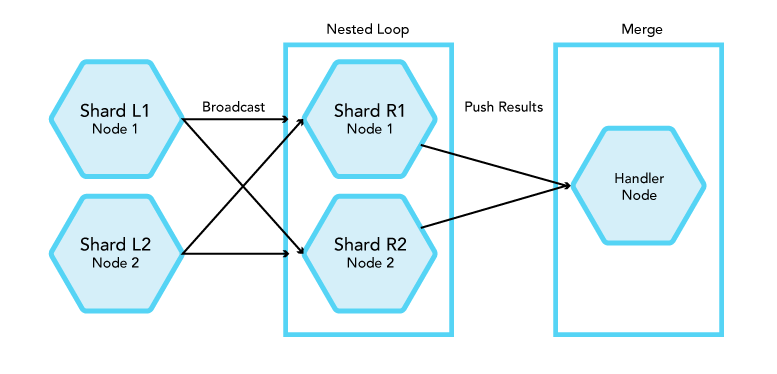Nodes that are holding the smaller shards broadcast the data to the processing nodes which then return the results to the requesting node.

Queries can be optimized if they contain (a) ORDER BY, (b) LIMIT, or (c) if INNER/EQUI JOIN. In any of these cases, the nested loop can be terminated earlier:

• Ordering allows determining whether there are records left

• Limit states the maximum number of rows that are returned

Consequently, the number of rows is significantly reduced allowing the operation to complete much faster.

### Hash join¶

The Hash Join algorithm is used to execute certain types of joins in a more efficient way than Nested Loop.

#### Basic algorithm¶

The operation takes place in one node (the handler node to which the client is connected). The rows of the left relation of the join are read and a hashing algorithm is applied on the fields of the relation which participate in the join condition. The hashing algorithm generates a hash value which is used to store every row of the left relation in the proper position in a hash table.

Then the rows of the right relation are read one-by-one and the same hashing algorithm is applied on the fields that participate in the join condition. The generated hash value is used to make a lookup in the hash table. If no entry is found, the row is skipped and the processing continues with the next row from the right relation. If an entry is found, the join condition is validated (handling hash collisions) and on successful validation the combined tuple of left and right relation is returned.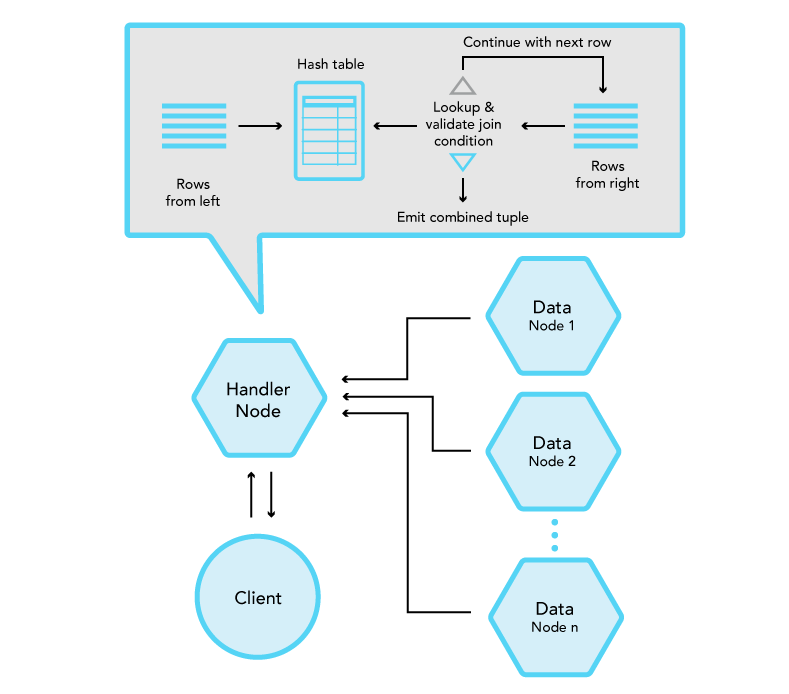Basic hash join algorithm

#### Block hash join¶

The Hash Join algorithm requires a hash table containing all the rows of the left relation to be stored in memory. Therefore, depending on the size of the relation (number of rows) and the size of each row, the size of this hash table might exceed the available memory of the node executing the hash join. To resolve this limitation the rows of the left relation are loaded into the hash table in blocks.

On every iteration the maximum available size of the hash table is calculated, based on the number of rows and size of each row of the table but also taking into account the available memory for query execution on the node. Once this block-size is calculated the rows of the left relation are processed and inserted into the hash table until the block-size is reached.

The operation then starts reading the rows of the right relation, process them one-by-one and performs the lookup and the join condition validation. Once all rows from the right relation are processed the hash table is re-initialized based on a new calculation of the block size and a new iteration starts until all rows of the left relation are processed.

With this algorithm the memory limitation is handled in expense of having to iterate over the rows of the right table multiple times, and it is the default algorithm used for Hash Join execution by CrateDB.

##### Switch tables optimization¶

Since the right table can be processed multiple times (number of rows from left / block-size) the right table should be the smaller (in number of rows) of the two relations participating in the join. Therefore, if originally the right relation is larger than the left the query planner performs a switch to take advantage of this detail and execute the hash join with better performance.

#### Distributed block hash join¶

Since CrateDB is a distributed database and a standard deployment consists of at least three nodes and in most case of much more, the Hash Join algorithm execution can be further optimized (performance-wise) by executing it in a distributed manner across the CrateDB cluster.

The idea is to have the hash join operation executing in multiple nodes of the cluster in parallel and then merge the intermediate results before returning them to the client.

A hashing algorithm is applied on every row of both the left and right relations. On the integer value generated by this hash, a modulo, by the number of nodes in the cluster, is applied and the resulting number defines the node to which this row should be sent. As a result each node of the cluster receives a subset of the whole data set which is ensured (by the hashing and modulo) to contain all candidate matching rows.

Each node in turn performs a block hash join on this subset and sends its result tuples to the handler node (where the client issued the query). Finally, the handler node receives those intermediate results, merges them and applies any pending `ORDER BY`, `LIMIT` and `OFFSET` and sends the final result to the client.

This algorithm is used by CrateDB for most cases of hash join execution except for joins on complex subqueries that contain `LIMIT` and/or `OFFSET`.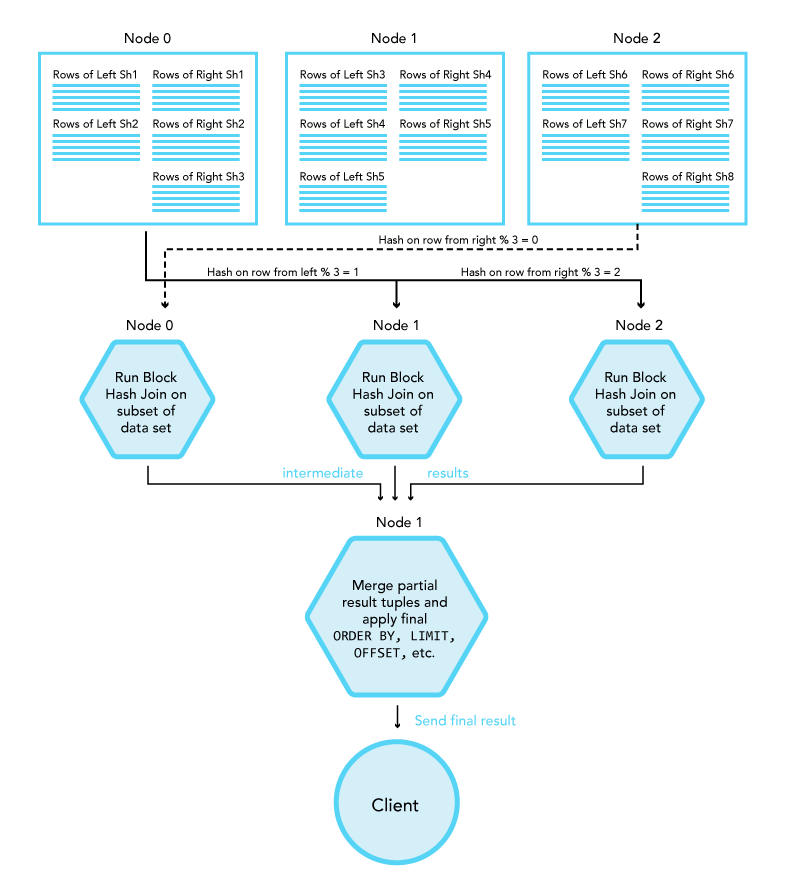Distributed hash join algorithm

## Join optimizations¶

### Query then fetch¶

Join operations on large relation can be extremely slow especially if the join is executed with a Nested Loop. - which means that the runtime complexity grows quadratically (O(n*m)). Specifically for cross joins this results in large amounts of data sent over the network and loaded into memory at the handler node. CrateDB reduces the volume of data transferred by employing “Query Then Fetch”: First, filtering and ordering are applied (if possible where the data is located) to obtain the required document IDs. Next, as soon as the final data set is ready, CrateDB fetches the selected fields and returns the data to the client.

### Push-down query optimization¶

Complex queries such as Listing 2 require the planner to decide when to filter, sort, and merge in order to efficiently execute the plan. In this case, the query would be split internally into subqueries before running the join. As shown in Figure 5, first filtering (and ordering) is applied to relations L and R on their shards, then the result is directly broadcast to the nodes running the join. Not only will this behavior reduce the number of rows to work with, it also distributes the workload among the nodes so that the (expensive) join operation can run faster.

```SELECT L.a, R.x
FROM L, R
WHERE L.id = R.id
AND L.b > 100
AND R.y < 10
ORDER BY L.a
```

Listing 2. An INNER JOIN on ids (effectively an EQUI JOIN) which can be optimized.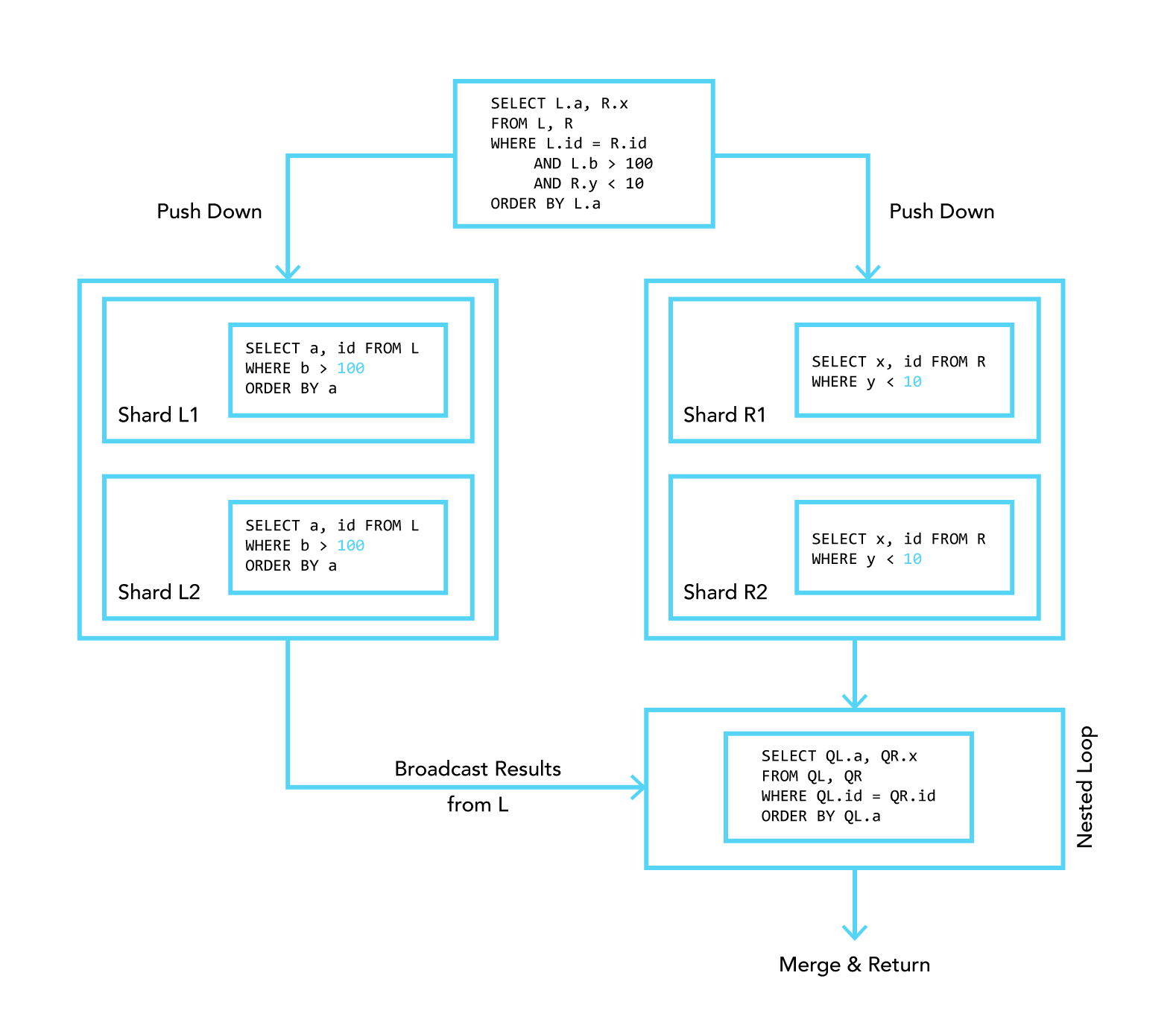Figure 5

Complex queries are broken down into subqueries that are run on their shards before joining.